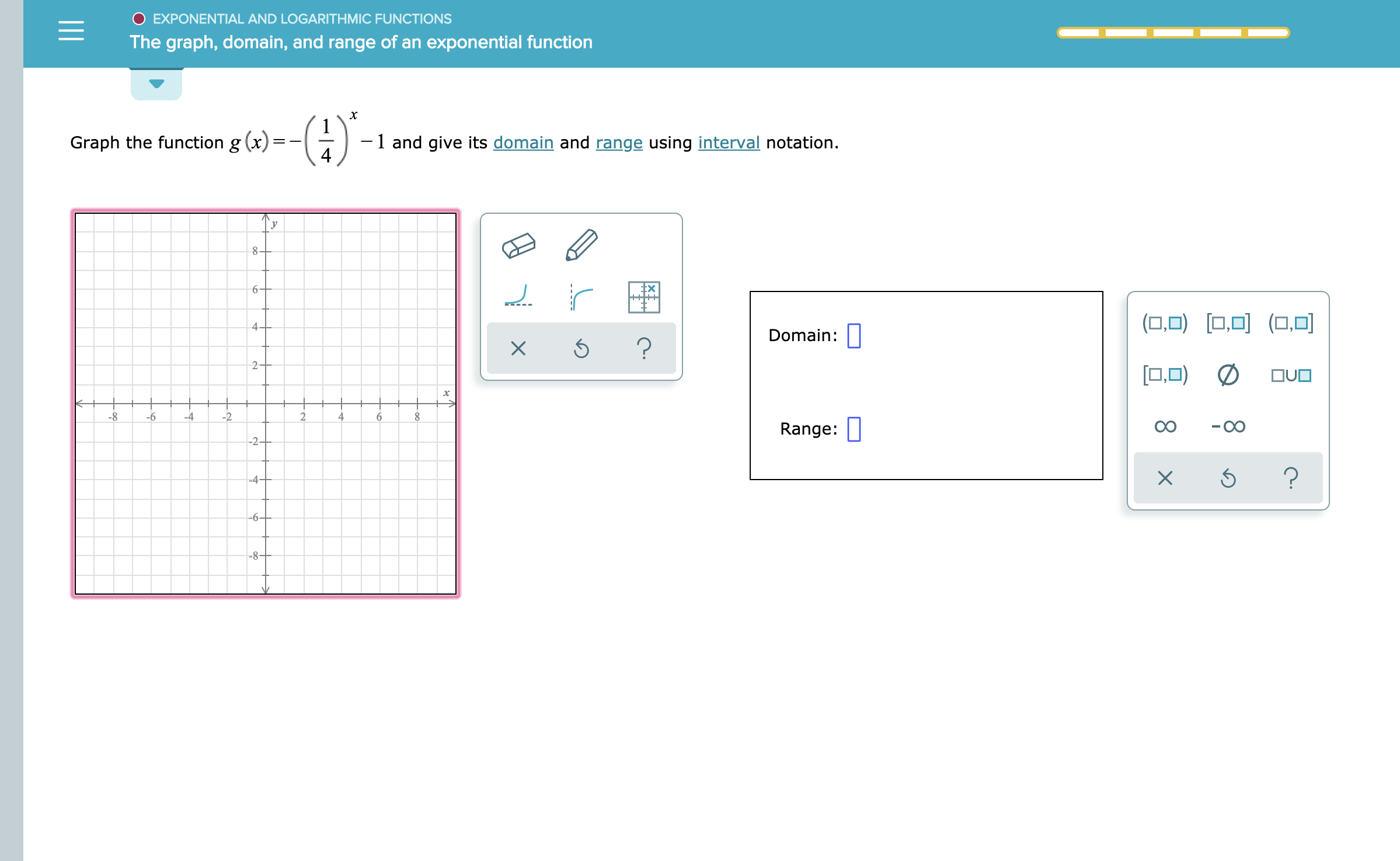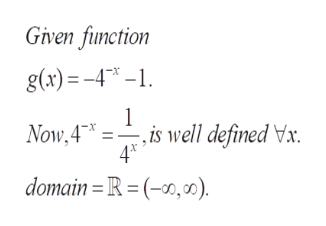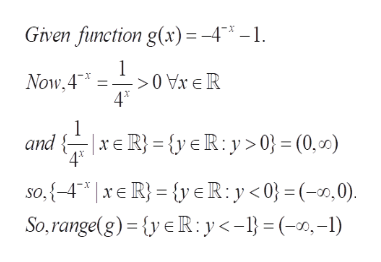# EXPONENTIAL AND LOGARITHMIC FUNCTIONSThe graph, domain, and range of an exponential function(})Graph the function g (x)=1 and give its domain and range using interval notation.6(O,O (ODomain:?20O)DOUO-8-6-224Range:-2--OO-4-?-6--8XX4

Question
28 views

see attachmenthelp_outlineImage TranscriptioncloseEXPONENTIAL AND LOGARITHMIC FUNCTIONS The graph, domain, and range of an exponential function (}) Graph the function g (x)= 1 and give its domain and range using interval notation. 6 (O,O (O Domain: ? 2 0O)D OUO -8 -6 -2 2 4 Range: -2- -OO -4- ? -6- -8 X X 4 fullscreen
check_circle

Step 1

To represent the given function graphically and identify its domain and range

Step 2

The function is well defined for all x , so the domain is the set of all real numbershelp_outlineImage TranscriptioncloseGiven function g(a)-4- 1 Now,4 4 * domain =R=(-10,0) x -,is well defined Vxr. fullscreen
Step 3

range of g = (- infinity,...help_outlineImage TranscriptioncloseGiven function g(x)= -4"*-1 1 Now,4 4* and R{yeR:y> = (0,0) so,{4* | xE R}= {{yeR:y0}=(-0, 0) So,range(g) {yeR: y < - = (-,-I) fullscreen

### Want to see the full answer?

See Solution

#### Want to see this answer and more?

Solutions are written by subject experts who are available 24/7. Questions are typically answered within 1 hour.*

See Solution
*Response times may vary by subject and question.
Tagged in

### Calculus